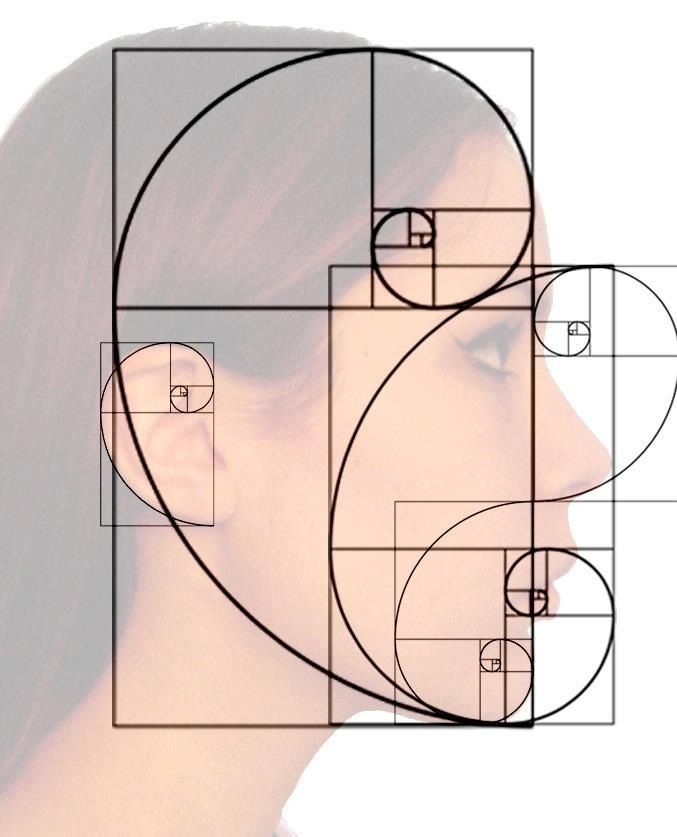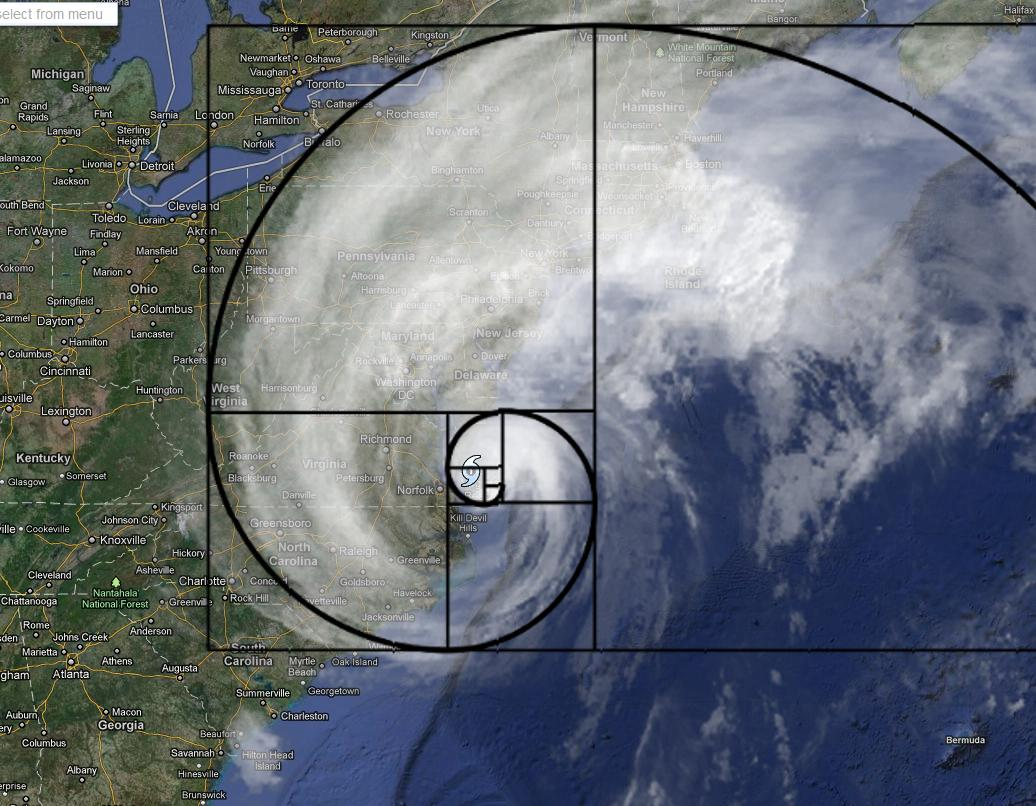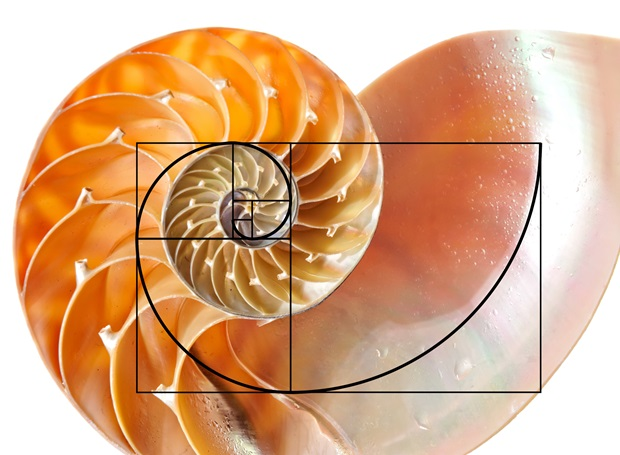June 19, 2018

## Understanding the Fibonacci SequenceYou have probably heard of the Fibonacci sequence, but do you know the historical context and practical trading applications behind these important numbers?

This article aims to shed a light on the mysterious Fibonacci sequence, and provide insights into some ways that traders can benefit from its use in market speculation.

#### History of the Fibonacci Sequence

The Fibonacci sequence is a set of numbers which are derived by adding the two numbers prior starting with 0 and 1.

This results in the following series of numbers:

0, 1, 1, 2, 3, 5, 8, 13, 21, 34, 55, 89, 144, 233, 377 and so on.

This number series is named after Leonardo Pisano Fibonacci, who first wrote about it in this 1202 book entitled “Liber Abaci”. He was a son of a merchant trader, and traveled extensively. It was in his travel to North Africa, where Fibonacci was introduced to the Ancient Hindu-Arabic number system. This was the inspiration behind Liber Abaci, and the roots for his passion and work in the development of mathematical concepts that ultimately led him to the Fibonacci sequence.

Oddly enough, Fibonacci first became aware of the sequence while he was working on a mathematical problem regarding the breeding habits of rabbits. The problem that he was trying to solve was the following:

“How many pairs of rabbits would be produced over a course of one year, beginning with a single pair, assuming that each pair bears a new pair every month, and the new pairs become productive beginning the second month.

As Fibonacci was pondering the solution, he eventually concluded that within a span of a year, 233 pairs of rabbits would be produced. And most notably the production cycle took on the following sequence:

0, 1, 1, 2, 3, 5, 8, 13, 21, 34, 55, 89, 144, 233

This was the origin of the Fibonacci sequence.

#### The Golden Ratio

The Fibonacci sequence provides us with the means to understand the Golden Ratio. The Golden Ratio is represented as Phi or 1.618, which is an irrational number and projects into infinite. As you divide any Fibonacci number by its previous number, the resulting number approaches Phi, represented by 1.618. Let’s take a few examples to clarify this:
Again, the Fibonacci numbers are listed below:

0, 1, 1, 2, 3, 5, 8, 13, 21, 34, 55, 89, 144, 233, 377…

Dividing 13 / 8 = 1.6250
Dividing 21 / 13 = 1.6153
Dividing 55 / 34 = 1.6176
Dividing 144 / 89 = 1.6179
Dividing 377 / 233 = 1.6180

The Golden Ratio can be applied to many different mathematical and geometric forms including Rectangles, Circles, Triangles, and others. Among the most prevalent formation that includes the Golden ratio is the Golden Spiral, which can be seen in many parts of the natural world. Some examples include flowers, plants, sea shells, and the spiraling galaxy.

#### Fibonacci Sequence in Nature

The Fibonacci Sequence is found in many different growth patterns in nature. Let’s take a closer look at few examples.

1. Storms and Hurricane – Many storm systems that can be seen on meteorological maps have a spiral shape. Just turn on the weather channel during the hurricane season, and you will see multiple instances of this.
2. Human Face – The human face is one of the most beautiful and symmetrical representation of the Fibonacci number sequence. Your face displays a high degree of Fibonacci proportions. From our ears, to our nose, to our mouth, there are numerous Fibonacci relationships that exist on the human face, and the entire human body for that matter.
3. Trees – Trees are everywhere around us. But have you ever taken the time to really study the amazing components of a tree? They display the most obvious characteristics of a fractal universe. The structure of trees are such that big branches grow out to form smaller branches, and smaller branches grow out to form even smaller branches, and so on and so forth.
4. Shells – Shells provide a beautiful illustration of Fibonacci numbers in nature. Shells follow a growth pattern that is unmistakably rooted in the Fibonacci sequence. The Nautilus shell is a perfect example of this.As we have seen, the Fibonacci sequence can be seen in many parts of our natural universe. And that leads into the final part of our discussion, which is on the topic of utilizing these numbers in the financial markets.

It is quite clear that the financial markets display a natural growth formation. Since all traders and investors in the markets are ultimately human beings, our emotions and reactions are part of the natural world. Our investment decisions are manifested on the price charts within a freely traded liquid market. And so, Fibonacci numbers can be seen in the markets. The astute trader can incorporate this information into making better trading decisions.

There are various Fibonacci tools available to the trader. Among them are Fibonacci Retracements, Fibonacci Extensions, Fibonacci Projections, Fibonacci Fans, and Fibonacci Time Zones. Although these tools all have their uses, the most practical and reliable tool among these is the Fibonacci Retracement tool.

Fibonacci retracements measure the depth of a correction from a prior impulsive move. The primary retracement levels include 38.2%, 50%, 61.8%, and 78.6%. Note that we have included 50% even though it is not part of the Fibonacci sequence. This is because 50% has special relevance in both mathematics and in trading.

Generally, traders would anticipate a reaction at these key retracement levels. Traders should study price action around these levels to gauge whether price is likely to find demand (buying interest) during a bearish correction, or find supply ( selling interest) during a bullish correction.

Since we know that Fibonacci numbers have a predictive value, it is considered a leading indicator rather than a lagging indicator such as traditional moving averages. As such we have the advantage of being able to position ourselves in advance in anticipation of a potential reaction.

Let’s take a look at an example of the Fibonacci Retracement tool on a price chart.This is the chart of Natural Gas (NG) from April 26 – May 10, 2018. Notice that prices started to head downward starting in late April. Soon after, the price of Natural Gas found some demand and price pushed higher until it reached the 78.6% retracement level. As soon as it hit this level, price dropped sharply on the reaction. Then price action made several pushes lower and began to stall and subsequently reversed to the upside.

The first test came at the 50% retracement level which rejected prices back to the downside. Shortly afterwards, prices came back to test the same 50% retracement level, and again swiftly pushed prices lower. There was one last attempt, but this time prices could not even reach the 50% level before dropping again.

Obviously not all levels will work out so nicely. But the point is that you should keep a keen eye
out for price action at key retracement levels, so that you are cognizant of opportunities that can provide for high probability trades.

Now that you have a better understanding and hopefully a deeper appreciation for the Fibonacci series, I would encourage you to learn more about the practical applications of Fibonacci within the framework of financial market speculation. It is a key arsenal in my trading toolbox and should be a consideration within the context of your own trading program.